# Fraction calculator

The calculator performs basic and advanced operations with fractions, expressions with fractions combined with integers, decimals, and mixed numbers. It also shows detailed step-by-step information about the fraction calculation procedure. Solve problems with two, three, or more fractions and numbers in one expression.

## Result:

### 21/2 - 1/4 = 9/4 = 2 1/4 = 2.25

Spelled result in words is nine quarters (or two and one quarter).

### How do you solve fractions step by step?

1. Conversion a mixed number 2 1/2 to a improper fraction: 2 1/2 = 2 1/2 = 2 · 2 + 1/2 = 4 + 1/2 = 5/2

To find a new numerator:
a) Multiply the whole number 2 by the denominator 2. Whole number 2 equally 2 * 2/2 = 4/2
b) Add the answer from previous step 4 to the numerator 1. New numerator is 4 + 1 = 5
c) Write a previous answer (new numerator 5) over the denominator 2.

Two and one half is five halfs
2. Subtract: 5/2 - 1/4 = 5 · 2/2 · 2 - 1/4 = 10/4 - 1/4 = 10 - 1/4 = 9/4
For adding, subtracting, and comparing fractions, it is suitable to adjust both fractions to a common (equal, identical) denominator. The common denominator you can calculate as the least common multiple of both denominators - LCM(2, 4) = 4. In practice, it is enough to find the common denominator (not necessarily the lowest) by multiplying the denominators: 2 × 4 = 8. In the following intermediate step, it cannot further simplify the fraction result by canceling.
In other words - five halfs minus one quarter = nine quarters.

#### Rules for expressions with fractions:

Fractions - simply use a forward slash between the numerator and denominator, i.e., for five-hundredths, enter 5/100. If you are using mixed numbers, be sure to leave a single space between the whole and fraction part.
The slash separates the numerator (number above a fraction line) and denominator (number below).

Mixed numerals (mixed fractions or mixed numbers) write as integer separated by one space and fraction i.e., 1 2/3 (having the same sign). An example of a negative mixed fraction: -5 1/2.
Because slash is both signs for fraction line and division, we recommended use colon (:) as the operator of division fractions i.e., 1/2 : 3.

Decimals (decimal numbers) enter with a decimal point . and they are automatically converted to fractions - i.e. 1.45.

The colon : and slash / is the symbol of division. Can be used to divide mixed numbers 1 2/3 : 4 3/8 or can be used for write complex fractions i.e. 1/2 : 1/3.
An asterisk * or × is the symbol for multiplication.
Plus + is addition, minus sign - is subtraction and ()[] is mathematical parentheses.
The exponentiation/power symbol is ^ - for example: (7/8-4/5)^2 = (7/8-4/5)2

#### Examples:

subtracting fractions: 2/3 - 1/2
multiplying fractions: 7/8 * 3/9
dividing Fractions: 1/2 : 3/4
exponentiation of fraction: 3/5^3
fractional exponents: 16 ^ 1/2
adding fractions and mixed numbers: 8/5 + 6 2/7
dividing integer and fraction: 5 ÷ 1/2
complex fractions: 5/8 : 2 2/3
decimal to fraction: 0.625
Fraction to Decimal: 1/4
Fraction to Percent: 1/8 %
comparing fractions: 1/4 2/3
multiplying a fraction by a whole number: 6 * 3/4
square root of a fraction: sqrt(1/16)
reducing or simplifying the fraction (simplification) - dividing the numerator and denominator of a fraction by the same non-zero number - equivalent fraction: 4/22
expression with brackets: 1/3 * (1/2 - 3 3/8)
compound fraction: 3/4 of 5/7
fractions multiple: 2/3 of 3/5
divide to find the quotient: 3/5 ÷ 2/3

The calculator follows well-known rules for order of operations. The most common mnemonics for remembering this order of operations are:
PEMDAS - Parentheses, Exponents, Multiplication, Division, Addition, Subtraction.
BEDMAS - Brackets, Exponents, Division, Multiplication, Addition, Subtraction
BODMAS - Brackets, Of or Order, Division, Multiplication, Addition, Subtraction.
GEMDAS - Grouping Symbols - brackets (){}, Exponents, Multiplication, Division, Addition, Subtraction.
Be careful, always do multiplication and division before addition and subtraction. Some operators (+ and -) and (* and /) has the same priority and then must evaluate from left to right.

## Fractions in word problems:

• Human 3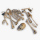Human bones are 3/10 living tissues 9/20 minerals, and the rest water. What fraction of human bone is water?
• Maria 3Maria spent two eighths of her holiday in Town A, two fifth in Town B and the rest on travelling. What fraction of her holiday did she spend on travelling?
• Pizza fractionsAnn ate a third of a pizza and then another quater. Total part of pizza eaten by Ann and how much pizza is left?
• Mountain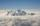Mountain has an elevation of 7450 meters and in the morning is the middle portion thereof in the clouds. How many meters of height is in the sky if below the clouds are 2,000 meters, and above clouds are two-fifths of the mountain's elevation?
• The pet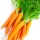Ananya has a bunny. She bought 4 7/8 pounds of carrots. She fed her bunny 1 1/4 pounds of carrots the first week. She fed her bunny 5/6 pounds of carrots the second week. All together, how many pounds of carrots did she feed her bunny? 1. Draw a tape diag
• Magic bagEach time the prince crossed the bridge, the number of tolars in the magic bag doubled. But then the devil always conjured 300 tolars for him. When this happened for the third time, the prince had twice as much as he had in the beginning. How many tolars
• Two ribbonsThe total length of the two ribbons is 13 meters. If one ribbon is 7 and 5/8 meters long, what is the length of the other ribbon?
• Plums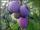In the bag was to total 136 plums. Igor took 3 plums and Mary took 4/7 from the rest. How many plums remained in the bag?
• Find the 24Find the difference between 2/7 and 1/21
• SchoolThere are 150 pupils in grade 5 . 2/3 of them are female. By what fractions are the males?
• Bitoo and ReenaBitoo ate 3/5 part of an apple and the remaining part was eaten by his sister Reena. How much part of an apple did Renna eat? Who had the larger share? By how much?
• Pizza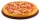Three siblings ordered one pizza. Miška ate a quarter of the whole pizza. Lenka ate a third of the rest and Patrik ate half of what Lenka had left. They had the rest packed up. How much of the pizza did they pack? Write the result as a fraction.
• RegroupingSubtract mixed number with regrouping: 11 17/20- 6 19/20